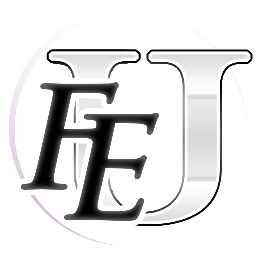Routine 01A370 determines whether an item can be equipped.

1. Return false if class = General and item = Levin Sword.
2. Return false if item = 0x00 (empty inventory slot)
3. Return false if item has the 0x01 item flag (Vulnerary, Silver Card, etc)
4. If item has a character lock: return true if user and lock match, return false if user and lock do not match. (Character Lock: Weapon Level = 0x80 + character number)
5. Return false if user’s weapon level < item’s weapon level.
6. Return false if user’s class is incompatible with the item’s type (explained below)
7. Return true, all the conditions have been passed.

Item type is determined by item number.
At 01A3E1 are the values that partition items into different types:
0C 11 16 1B 20 2B 36 37 40

``````        X < 0x0C : type = 0 (sword)
0x0C <= X < 0x11 : type = 1 (lance)
0x11 <= X < 0x16 : type = 2 (bow)
0x16 <= X < 0x1B : type = 3 (ballista)
0x1B <= X < 0x20 : type = 4 (axe)
0x20 <= X < 0x2B : type = 5 (dragonstone)
0x2B <= X < 0x36 : type = 6 (tome)
0x36 <= X < 0x37 : type = 7 (staff A; Heal staff)
0x37 <= X < 0x40 : type = 8 (staff B; all other staves)
``````

At 01A3EA is a pointer table to class lists.
Use type number as an index to find the classes that are compatible with the item type.

It’s interesting that staves are split into 2 groups. Perhaps they were planning to have a class that could use the basic Heal staff, but not any of the other staves (maybe Mage?)

Adding additional weapons (like replacing an unused dragonstone with a Steel Lance) would be a bit troublesome. You would have to rearrange item data (which is spread across multiple tables) to make sure all items of the same type stay grouped together. Also, you might break other things that depend on item number. Yeah, let’s just have a table that defines item type.

Paste this at 03F9A0 (item type table)

``````00 00 00 00 00 00 00 00 00 00 00 01 01 01 01 01 02 02 02 02 02 03 03 03 03 03 04 04 04 04 04 05 05 05 05 05 05 05 05 05 05 05 06 06 06 06 06 06 06 06 06 06 06 07 08 08 08 08 08 08 08 08 08 09 09 09 09 09 09 09 09 09 09 09 09 09 09 09 09 09 09 09 09 09 09 09 09 09 09 09 09 09
``````

Paste this at 01A3A6 (code to read the table)

``````BD 90 F9 C9 09 B0 2B EA EA EA EA EA EA EA EA EA EA
``````

Here’s a Nightmare module to edit the new item type table:

[spoiler=FE1 Item Type Editor]1
FE1 Item Type Editor
0x3F9A0
92
1
Item_list.txt
NULL

Item Type
0
1
NDHU
Item Type.txt[/spoiler]
[spoiler=Item Type]10
0x00 Sword
0x01 Lance
0x02 Bow
0x03 Ballista
0x04 Axe
0x05 Dragonstone
0x06 Tome
0x07 Staff A
0x08 Staff B
0x09 Can’t Equip[/spoiler]

Also, don’t forget to get the new item module and set the range/usability byte so the weapon can actually attack.

Finally, you need to set the name of the new weapon.
Item name pointer table located at 03DAE5. (NES pointers are weird. When viewing data in a hex editor you need to add 0x10 to the pointer to get the correct address)

[spoiler=FE1 Item Name Pointers]1
FE1 Item Name Editor
0x3DAE5
92
2
Item_list.txt
NULL

Pointer
0
2
NEHU
NULL[/spoiler]

Here’s a table file for the basic characters in the Quirino translation patch:
[spoiler=Text table]45=a
46=b
47=c
48=d
49=e
4A=f
4B=g
4C=h
4D=i
4E=j
4F=’
50=k
51=l
52=m
53=n
54=o
55=p
56=q
57=r
58=s
59=t
5A=u
5B=v
5C=w
5D=x
5E=y
5F=z
60=0
61=1
62=2
63=3
64=4
65=5
66=6
67=7
68=8
69=9
6A=A
6B=B
6C=C
6D=D
6E=E
6F=F
70=G
71=H
72=I
73=J
74=K
75=L
76=M
77=N
78=O
79=P
7A=Q
7B=R
7C=S
7D=T
7E=U
7F=V
80=W
81=X
82=Y
83=Z[/spoiler]

And here are the item symbols:
[spoiler=Item symbols]84 = Sword
85 = Lance
86 = Bow
87 = Axe
88 = Key
89 = Tome
8A = Staff
8B = Ballista
96 = Dragonstone
97 = Orb
98 = Medal
99 = Ring
9A = Potion
A7 = Falchion
A8 = Card[/spoiler]

Item name terminated with 0xEF.(Replaced the unused Wyvern Stone and Gargoyle Stone with a Steel Lance and Silver Axe)

3 Likes